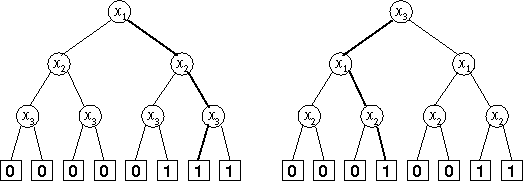# S-Trees

Time Limit: 1000MS

Memory Limit: 10000K

## Description

A Strange Tree (S-tree) over the variable set Xn = {x1,x2,...,xn} is a binary tree representing a Boolean function f:{0,1}->{0,1}. Each path of the S-tree begins at the root node and consists of n+1 nodes. Each of the S-tree's nodes has a depth, which is the amount of nodes between itself and the root (so the root has depth 0). The nodes with depth less than n are called non-terminal nodes. All non-terminal nodes have two children: the right child and the left child. Each non-terminal node is marked with some variable xi from the variable set Xn. All non-terminal nodes with the same depth are marked with the same variable, and non-terminal nodes with different depth are marked with different variables. So, there is a unique variable xi1 corresponding to the root, a unique variable xi2 corresponding to the nodes with depth 1, and so on. The sequence of the variables xi1,xi2,...,xin is called the variable ordering. The nodes having depth n are called terminal nodes. They have no children and are marked with either 0 or 1. Note that the variable ordering and the distribution of 0's and 1's on terminal nodes are sufficient to completely describe an S-tree. As stated earlier, each S-tree represents a Boolean function f. If you have an S-tree and values for the variables x1,x2,...,xn, then it is quite simple to find out what f(x1,x2,...,xn) is: start with the root. Now repeat the following: if the node you are at is labelled with a variable xi, then depending on whether the value of the variable is 1 or 0, you go its right or left child, respectively. Once you reach a terminal node, its label gives the value of the function.Figure 1: S-trees for the x1 and (x2 or x3) function
On the picture, two S-trees representing the same Boolean function,f(x1,x2,x3) = x1 and (x2 or x3), are shown. For the left tree, the variable ordering is x1, x2, x3, and for the right tree it is x3, x1, x2. The values of the variables x1,x2,...,xn, are given as a Variable Values Assignment (VVA)
(x1 = b1, x2 = b2, ..., xn = bn)
with b1,b2,...,bn in {0,1}. For instance, ( x1 = 1, x2 = 1 x3 = 0) would be a valid VVA for n = 3, resulting for the sample function above in the value f(1,1,0) = 1 and (1 or 0) = 1. The corresponding paths are shown bold in the picture. Your task is to write a program which takes an S-tree and some VVAs and computes f(x1,x2,...,xn) as described above.

## Input

The input contains the description of several S-trees with associated VVAs which you have to process. Each description begins with a line containing a single integer n, 1 <= n <= 7, the depth of the S-tree. This is followed by a line describing the variable ordering of the S-tree. The format of that line is xi1 xi2 ...xin. (There will be exactly n different space-separated strings). So, for n = 3 and the variable ordering x3, x1, x2, this line would look as follows: x3 x1 x2 In the next line the distribution of 0's and 1's over the terminal nodes is given. There will be exactly 2^n characters (each of which can be 0 or 1), followed by the new-line character. The characters are given in the order in which they appear in the S-tree, the first character corresponds to the leftmost terminal node of the S-tree, the last one to its rightmost terminal node. The next line contains a single integer m, the number of VVAs, followed by m lines describing them. Each of the m lines contains exactly n characters (each of which can be 0 or 1), followed by a new-line character. Regardless of the variable ordering of the S-tree, the first character always describes the value of x1, the second character describes the value of x2, and so on. So, the line 110 corresponds to the VVA ( x1 = 1, x2 = 1, x3 = 0). The input is terminated by a test case starting with n = 0. This test case should not be processed.

## Output

For each S-tree, output the line "S-Tree #j:", where j is the number of the S-tree. Then print a line that contains the value of f(x1,x2,...,xn) for each of the given m VVAs, where f is the function defined by the S-tree. Output a blank line after each test case.

## Sample Input

3
x1 x2 x3
00000111
4
000
010
111
110
3
x3 x1 x2
00010011
4
000
010
111
110
0

## Sample Output

S-Tree #1:
0011

S-Tree #2:
0011

## Source

Mid-Central European Regional Contest 1999